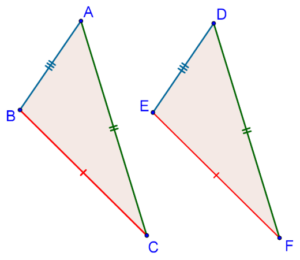# The SSS Criterion

Go back to  'Triangles'

Consider the following two triangles, for which the corresponding sides are equal:That is, it is given to us that AB = DE, AC = DF and BC = EF. Will the two triangles be congruent?

Let us look at this question from another perspective. Suppose that you are given the lengths of the three sides of a triangle. How many different triangles can you draw with these three lengths? Can you draw more than one triangle? The answer is: No, you can draw exactly one triangle, given the lengths of its sides. This means that the lengths of a triangle’s sides uniquely determine it. In other words, if two triangles have the same set of lengths, then they must be congruent. Can we say the same if only two pairs of (corresponding) sides are equal in two triangles? Of course not! Given two sides, infinitely many triangles can be drawn. Thus, there will be no such thing as an SS congruence criterion.

Before we go on to the proof of the SSS criterion, here is a useful activity which you are strongly urged to perform:

1. Take three lengths; say 5 cm, 6 cm and 7 cm. Now, geometrically construct a triangle with these sides. And most importantly, convince yourself that exactly one such triangle can be drawn.

2. Now, take only two lengths, say 5 cm and 6 cm. Construct three different triangles with these as two of their sides. Going further, try to understand that once the third side is fixed, the triangle itself will be fixed (unique).

grade 9 | Questions Set 1